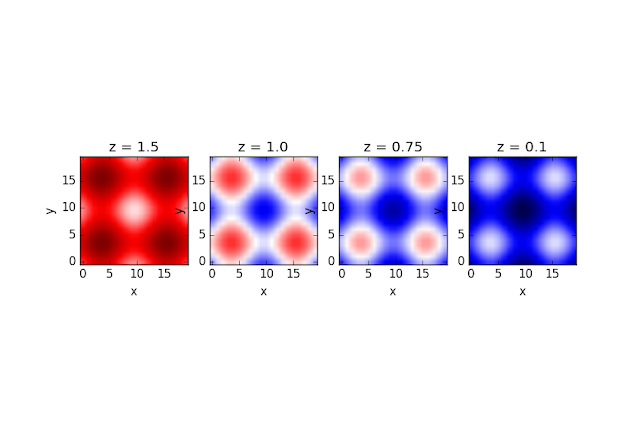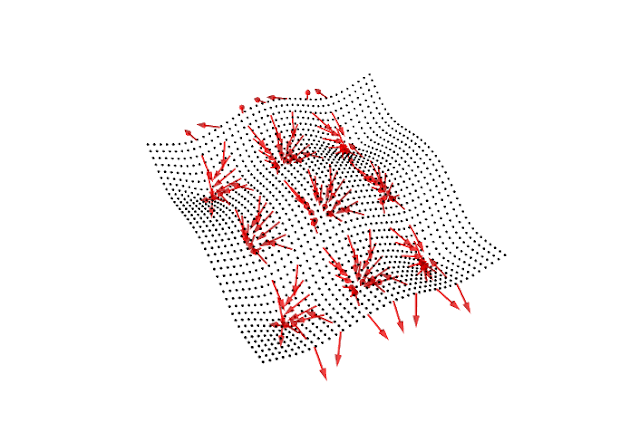## Saturday, November 4, 2017

### BFGS - part 1

Nothing to do with Roald Dahl but a trick used to optimize machine learning algorithms (see Spark's mllib library). We're iteratively trying to find the lowest point in some space and representing this value with mk where k is the iteration step number.

mk = f(xk) + ∇ f(xk) T pk + (pkT Bk pk) / 2

taken from a Taylor expansion where pk is the step and Bk is a Hessian matrix that is positive definite (for reasons concerning convergence - see below).

At the minimum, ∇mk will equal 0. Also, the last term will disappear (since our model is quadratic). Re-arranging gives:

pk = - Bk-1 ∇ f(xk)

So, the next step can be given as:

xk+1 = xk + αkBk-1 ∇ f(xk)

where αk is the step size at the k-th step.

Why positive definite?

A positive definite matrix, M, satisfies xTM x > 0 for all x. Note that if we do a Taylor expansion around the minimum point x*, we get

f(x* + p) = f(x*) + pT∇ f(x*) + pT ∇2 f(x*p / 2 + ...

where obviously ∇ f(x*) is zero and f(x*) is less than f(x* + p) by the definition of x*. Therefore, the final term is greater than 0 and hence it is positive definite. QED.

See p16 of Numerical Optimization (Norcedel & Wright) for a more convincing argument.

Surfaces

We can treat the space just like an electromagnetic field that has an electric potential at every point in that space. So, although there is a scalar associated with every point in that space, how that scalar changes can lead to a vector associated with every point in that space.

Lets' take an example (full source code available here):

import mayavi.mlab as plt
import numpy as np
import math as m
from sympy import *

x, y, z = symbols('x y z')

f = z ** 2 * cos(x) ** 2 +  cos(y) ** 2

what does this look like? Well, since it's a scalar value at every point in 3-D space, it's hard to visualize. We could view it as a heatmap at various values of z (that is, take a slice on the x-y plane for a given z value).Slices of the space of f at given values of z
Or we could fix f for an arbitrary value and look at the surface that is defined by all points that map to that value (an "equipotential" in physics terms). For instance:Surfaces at f=0 (bottom), f=2 (middle) and f=4 (top)
For simplicity, let's arbitrarily look at the surface formed by f=0 and look at the gradient - that is ∇f(xk) - along the line x=0 that we chose arbitrarily.

Because I'm a careless mathematician, it's a pleasure to find that Sympy does the work for me. I write:

div = [f.diff(v) for v in [x, y, z]]

then I can calculate the gradient vector at all points on this surface with:

gradx = div.subs(x, xpt).subs(y, ypt).subs(z, zpt).evalf()
grady = div.subs(x, xpt).subs(y, ypt).subs(z, zpt).evalf()
gradz = div.subs(x, xpt).subs(y, ypt).subs(z, zpt).evalf()

and plot it so:gradient where f=0 and x=0
Unsurprisingly, the gradient vectors are perpendicular to the surface.

Now we add the vectors corresponding to Bk-1 ∇ f(xk) along this line of x=0. Again, Sympy does all the heavy lifting:

def eval_p_at(xpt, ypt, zpt):
co_ords = [xpt, ypt, zpt]
hessian_fn = [[f.diff(a).diff(b) for a in [x, y, z]] for b in [x, y, z]]
values = [sub_vals(item, co_ords) for item in hessian_fn]
hess_mat_eval = Matrix(values)
div_vals = sub_vals(div, co_ords)
if hess_mat_eval.det() == 0:
print "uninvertible hessian at ", xpt, ypt, zpt
return 0, 0, 0
else:
p = - (hess_mat_eval.inv() * Matrix(div_vals))
return float(p), float(p), float(p)

def sub_vals(items, vals):

return [i.subs(x, vals).subs(y, vals).subs(z, vals).evalf() for i in items]

where this is the equation for p we see at the top of this post. Plotting it gives:Gradient and p along f=0, x=0
Note how the p vectors are all pointing downwards, trying to minimize f.

Now, if we look at these vectors of p at varies points on this arbitrarily chosen surface, f=0, it looks like this:p vectors on f=0
which is starting to look like our heatmap if we looked directly down on it. The p vectors are trying to find the "coldest" spot, the minimum of the function f.

I'll flesh out the algorithm more in the next post.